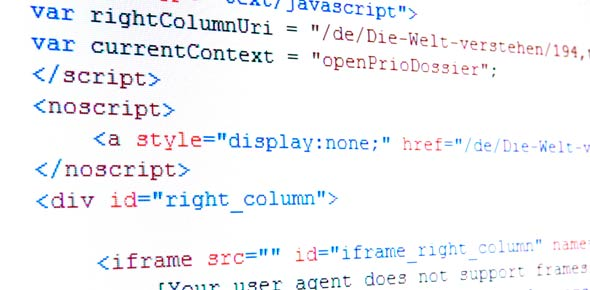# Programming Quiz For Sjc Seniors

15 Questions | Total Attempts: 144SettingsHere's a quick quiz to assess your knowledge on intro to programming. It is scheduled for 10 minutes. Enter your Name and Class in the Name box and click the start button to commence with your quiz. When you are finished with the quiz, check out how you did and the correct answers. Good Luck!

• 1.
Algorithms have four very important attributes; what are they?
• A.

Simple, Conditional, Loop, Arrays

• B.

Precise, Unambiguous, Finite, Logical Sequence

• C.

Define problem, find solution, evaluate alternate solution, check correctness

• D.

None of the above

• 2.
The design of any computer program involves two major phases; they are:
• A.

Define problem and write algorithm

• B.

Translate algorithm into language and Compile

• C.

Problem Solving and Program Implementation

• D.

None of the above

• 3.
A defining diagram comprises of three sections; they are:
• A.

• B.

Simple, Conditional, Loop

• C.

Input, Processing, Output

• D.

None of the above

• 4.
Which is NOT true of a variable?
• A.

Can be a minimum of 40 characters

• B.

Cannot have spaces

• C.

• D.

None of the above

• 5.
A variable is:
• A.

A small block of memory that stores a value

• B.

Something within an algorithm that can change

• C.

Usually referred to as a constant

• D.

None of the above

• 6.
A ___________ is an area of storage whose value never changes during processing?
• A.

Variable

• B.

Algorithm

• C.

Constant

• D.

Literal

• 7.
Which of the following is an algorithm whereby a segment of the code is repeated based on a condition?
• A.

Simple

• B.

Conditional

• C.

Loop

• D.

Array

• 8.
Within the following segment of a pseudocode algorithm, which line number represents the Assignment Statement? 1. READ num1, num2, num32. LET Total = num1+num2+num33. PRINT Total
• A.

1

• B.

2

• C.

3

• D.

None of the above

• 9.
Which of the following is used within a flowchart to represent a decision point?
• A.

Parallelogram

• B.

Rectangle

• C.

Diamond

• D.

None of the above

• 10.
The following is the syntax for which algorithm?Input Let = Print ,
• A.

Simple

• B.

Conditional

• C.

Loop

• D.

None of the above

• 11.
An assembler is used to convert which generation of computer language back to machine code?
• A.

First Generation

• B.

Second Generation

• C.

Third Generation

• D.

Fourth Generation

• 12.
PASCAL is an example of what TYPE of programming language?
• A.

4th Generation Language

• B.

Scripted Language

• C.

Structured Language

• D.

3rd Generation Language

• 13.
The Computer Language that uses binary is:
• A.

Assembler Language

• B.

Assembly Language

• C.

Machine Language

• D.

None of the above

• 14.
The program that translates high level programs into machine code is known as:
• A.

Complier

• B.

Assembler

• C.

Pascal

• D.

None of the above

• 15.
An example of a dry run is:
• A.

Algorithm

• B.

Pascal

• C.

Pseudocode

• D.

All of the above

Related TopicsBack to top